# Team:ZJU-China/Model/second

Infiltration Model
Infiltration

Background

In our experiment, we have designed and performed the microfluidic devices(Pic 1), where the substrate inside wash the increasing AHL in the chamber room. On the one hand, we controlled the concentration of AHL in the chamber room, on the other hand, the diffusion of AHL realized the united oscillation among each chamber.
Therefore, we constructed a model on the relationship between the flow rate of microfluidic and the concentration of AHL.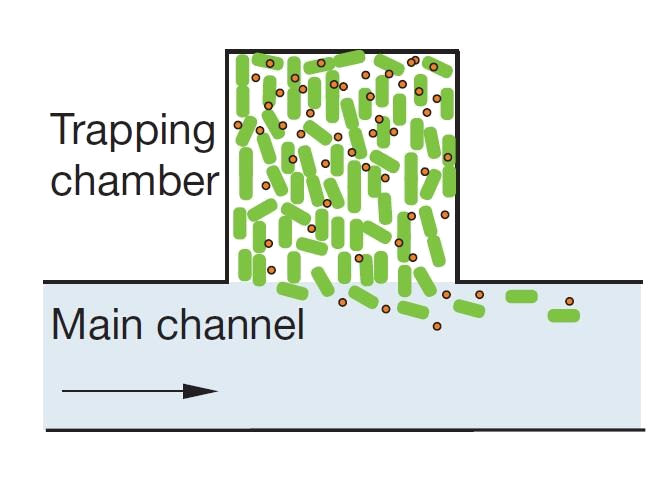Pic 1 Microfluidic Device
Derivation

When the microfluidic substrates flows across the chamber room, the external AHL molecule in the chamber room penetrates from the surface towards the main body. At every instant of the system, there exists different instant distribution of concentration and instant interface diffusion rate, which is proportional to the concentration gradient of the interface. Thereunto, the entire process of mass transformation by convection is achieved by to different effects.
(1) Convective transformation effect. That is, under the circumstance of convection, the fluid mass point continually moves and confuses, and brings the substance from one place to another.
(2) Molecule Diffusion Effect. It is caused by the concentration difference among the fluid, the molecule transfers by the means of diffusion.
Mass transformation coefficient DAB is the combined signal of two effects, whose unit is m^2/s.
According to Higbie’s penetration model , when composite A diffuses towards composite B, the mass flux through the interface of two liquid phases can be presented as: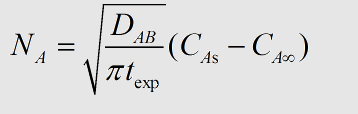Where texp is the exposure time of two liquid phases, CAs is the concentration of AHL of the higher concentration side of the interface, and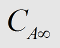is the concentration of the lower side. In our model, the input microfluidic doesn’t contain AHL. Besides, we neglected the effect that the outside AHL make to the inside AHL. Thus, we can make the assumption that=0.
After the integration of the equation mentioned above, during the exposure time of texp, the total solute which penetrated to the vortex is: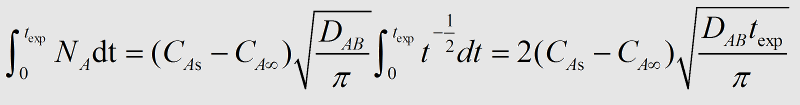During the explosion time, the average mass transfer flux is: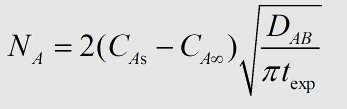In our model,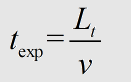.
Where Lt is the efficient length where the chamber room and the microfluidic contacts, 100um. v is the flow rate of the microfluidic. Then we can get :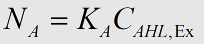, where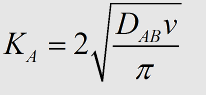.
Simulated Resluts

Therefore, we get the data from some literature and our experiments that DAB=2.5. Besides, we constructed our modeling analysis about the flow rate v and the initial concentration of outside AHL molecules CA, we can get the following figures: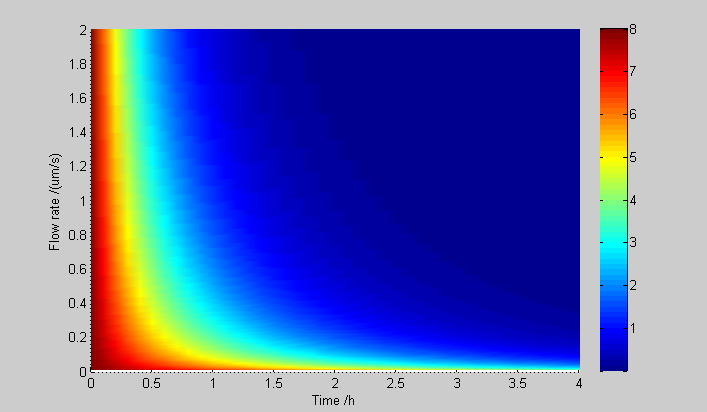Pic 2 Effect of different flow rate to the Microfluidic Device with a given initial concentration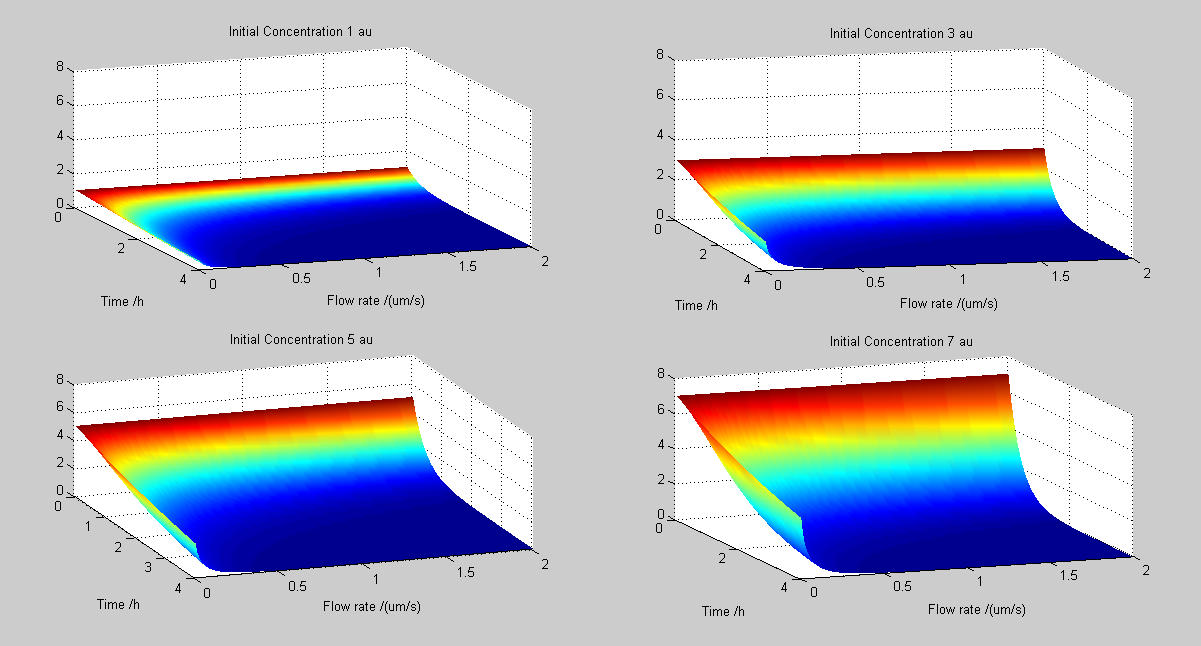Pic 3 Effect of different flow rate to the Microfluidic Device with different initial concentrations